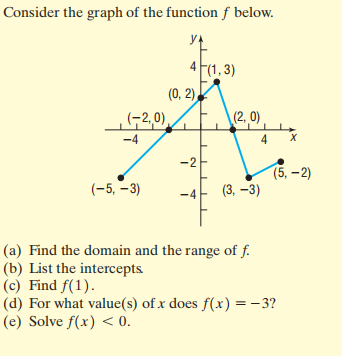### Still have math questions?

Algebra
QuestionConsider the graph of the function $$f$$ below.

(a) Find the domain and the range of $$f$$ .

(b) List the intercepts (c) Find $$f ( 1 )$$ .

(d) For what value(s) of $$x$$ does $$f ( x ) = - 3$$ ?

(e) Solve $$f ( x ) < 0$$ .

$$\text { (a) Domain: } \{ x | - 5 \leq x \leq 5 \} \text { ; range: } \{ y | - 3 \leq y \leq 3 \} \quad \text { (b) } ( 0,2 ) , ( - 2,0 ) \text { , and } ( 2,0 ) \quad \text { (c) } f ( 1 ) = 3 \quad \text { (d) } x = - 5 \text { and } x = 3$$